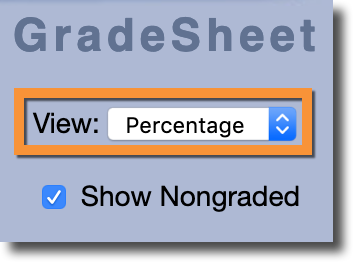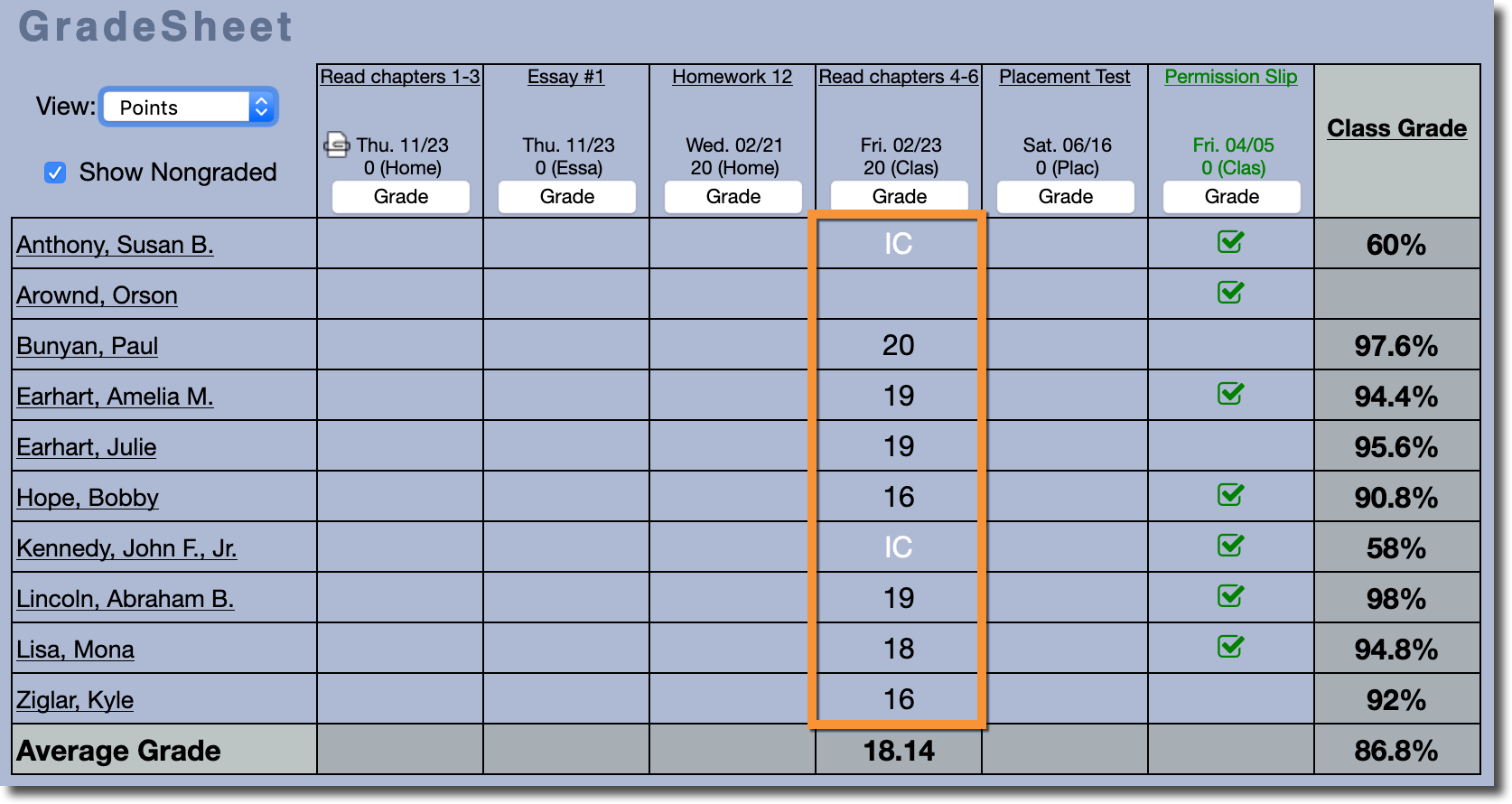Scenario:

Solution:

1. Convert any Non-Percentage Grades into Percentages.
2. Add the Weight of each Assignment together.
3. Multiply the Percentage Grade for each Assignment by its respective Weight.
4. Add the values calculated in Step 3 together.
5. Divide the number calculated in Step 4 by the number calculated in Step 2.

Let's look at this process in more detail using the following grade sheet as an example. We'll be looking specifically at the student Susan B. Anthony.### Step 1 - Convert any Non-Percentage Grades into PercentagesIf we switch to the "Points" View, we can see that one of the assignments was actually graded using points and those grades were being converted into percentages.There are two types of grades that are not converted into Percentages: Assignment Grade Codes and Non-Graded Assignments.

• Assignment Grade Codes are built-in to Gradelink and include non-traditional grades such as "Incomplete" and "Excused". By default, the only assignment grade code that can influence the class grade is "Incomplete", which converts to 0%. However, this can adjusted by schools if they wish.
• Non-Graded Assignments will never impact the class grade under any circumstances.

### Step 2 - Add the Weight of each Assignment together

The next step in calculating a class grade is to calculate the "Total Weight" by adding together the Weights associated with each assignment.

Before Teachers can add assignments to their classes, they must create "Assignment Types". Assignment Types allocate a specific percentage, or 'weight', of the overall class grade to specific types of assignments. For example, a Teacher may want to guarantee that Tests make up 30% of a student's grade.

When an assignment is created, it is given an assignment type. As more assignments use the same assignment type, the 'weight' of each individual assignment goes down. For example, if the assignment type "Homework" is worth 20% of the class grade, and there are 4 Homework assignments, each one will have a weight of 5% (20% divided by 4 assignments). If the Teacher added a fifth Homework assignment, then each would be worth 4% (20% divided by 5 assignments).

On the grade sheet, you can view the current weight for each assignment by switching to the Percentage view and looking at the numbers that appear above the "Grade" button.However, just because an assignment has a weight associated with it does not mean it affects a student's class grade. In the example above, we can see that no grade has been entered for one assignment (the blank space in the third column).

When no grade is entered for an assignment, that assignment will not be counted when calculating a student's class grade even if the assignment has a weight associated with it.

Note: Assignments are still weighted even if they aren't graded. Therefore, even if it isn't graded, an assignment can still impact a Student's grade.

Therefore, in the example above the Total Weight is 37.5% (7.5% + 15% + 15% + 0%).

### Step 3 - Multiply the Percentage Grade for each Assignment by its respective Weight

The next step in calculating a class grade is multiplying the grade a student has received in each assignment by its respective weight.

The student in the example shown above received 100% on an assignment with a weight of 7.5%. Multiplying these numbers together results in a value of 7.50%. Repeat this process for every assignment where the student received a grade.